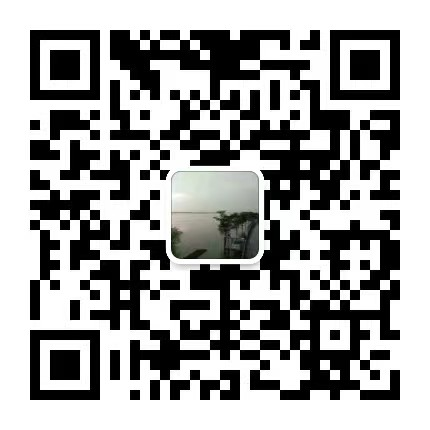## 2021年陕西成考高升本层次数学模拟题（一）

2021-03-27 09:49:18   来源：admin    点击： 0 微信咨询+ 加入微信交流群+ 加入1.设集合A={a,b,c,d,e}  B={a,b,e},则AUB=(            )
A {a,b,e }  B {c,d} C {a,b,c,d,e}  D2.下列函数为偶函数的是（　　　）
Ay=-x  B y=xsinx  C y=xcosx  D y=x2+x
3.条件甲x=2,条件乙：x2-3x+2=0，则条件甲是条件乙的（　　　）
A　充要条件　　B必要不充分条件　　C充分但不必条件　　D既不充分又不必要条件
4.到两定点A（-1,1）和B（3,5）距离相等的点的轨迹方程为（　　　）
A x+y-4=0  B x+y-5=0  C x+y+5=0  D x-y+2=0
5.两条平行直线z1=3x+4y-5=0与Z2=6x+8y+5=0之间的距离是（　　　）
A 2   B 3   C 2(1)  D 2(3)
6.以椭圆16(x2)+9(y2)=1上的任意一点（长轴两端除外）和两个焦点为顶点的三角形的周长等于（　）
A 12   B 8+2  C 13   D 18
7.函数y=的定义域是（　　）
A  R   B[0,+∞]    C[-4,-2]   D(-4,-2)
8.抛物线y2=-4x上一点P到焦点的距离为3,则它的横坐标是（　　　）
A -4   B -3   C -2   D -1
9.函数f(x)=sinx+x3(   )
A是偶函数　　B是奇函数　　C既是奇函数，又是偶函数　　D既不是奇函数也不是偶函数
10.=(　　　)
A4(1)   B 2(1)   C 2(3 )   D 4(3 )
11.掷两枚硬币，两枚的币值面都朝上的概率是（　　　）
A 2(1)   B 4(1)   C 3(1)   D 8(1)
12.通过点（3,1）且与直线x+y=1垂直的直线方程是（　　　）
A x-y+2=0   B 3x-y-8=0   Cx-3y+2=0   Dx-y-2=0
13.已知y=loga(2-ax)在［0,1］上是x的减函数，则a的取值范围是（　　　）
A 9(1)   B (1,2)   C (0,2)   D (2,+ ∞)
14.如果向量a=(3,-2),b=(-1,2)，则(2a+b)·(a-b)等于（　　　）
A 28   B 8   C 16   D32
15.若从一批有8件正品，2件次品组成的产品中接连抽取2件产品（第一次抽出的产品不放回去），则第一次取得次品且第二次取得正品的概率是（　　　）
A 9(1)  B 9(2)   C 45(8)   D 45(16)

### 关注公众号### 考试提醒

2023年全国成人高考还有

00